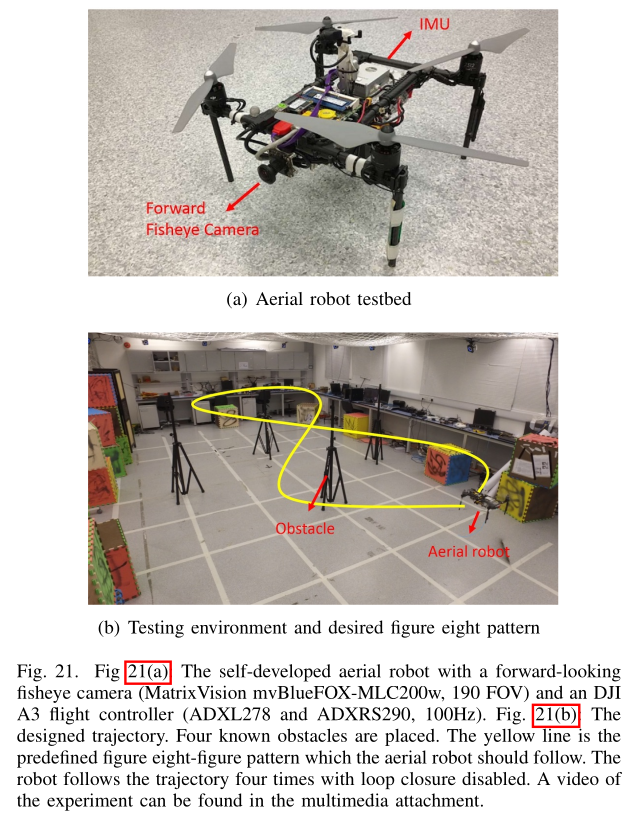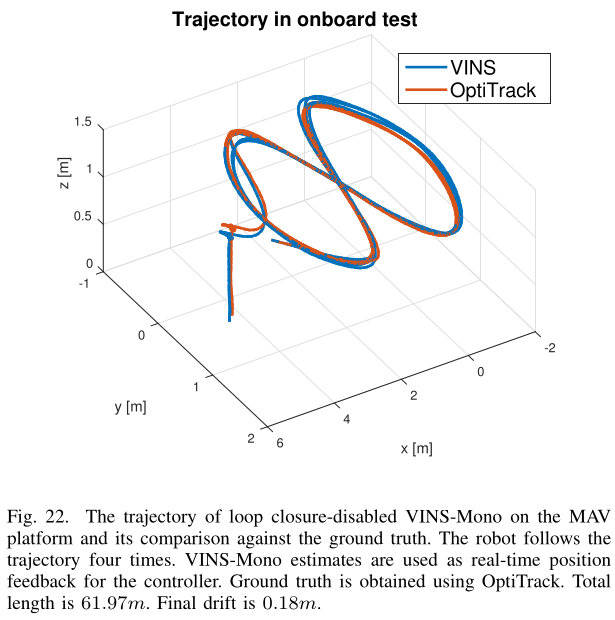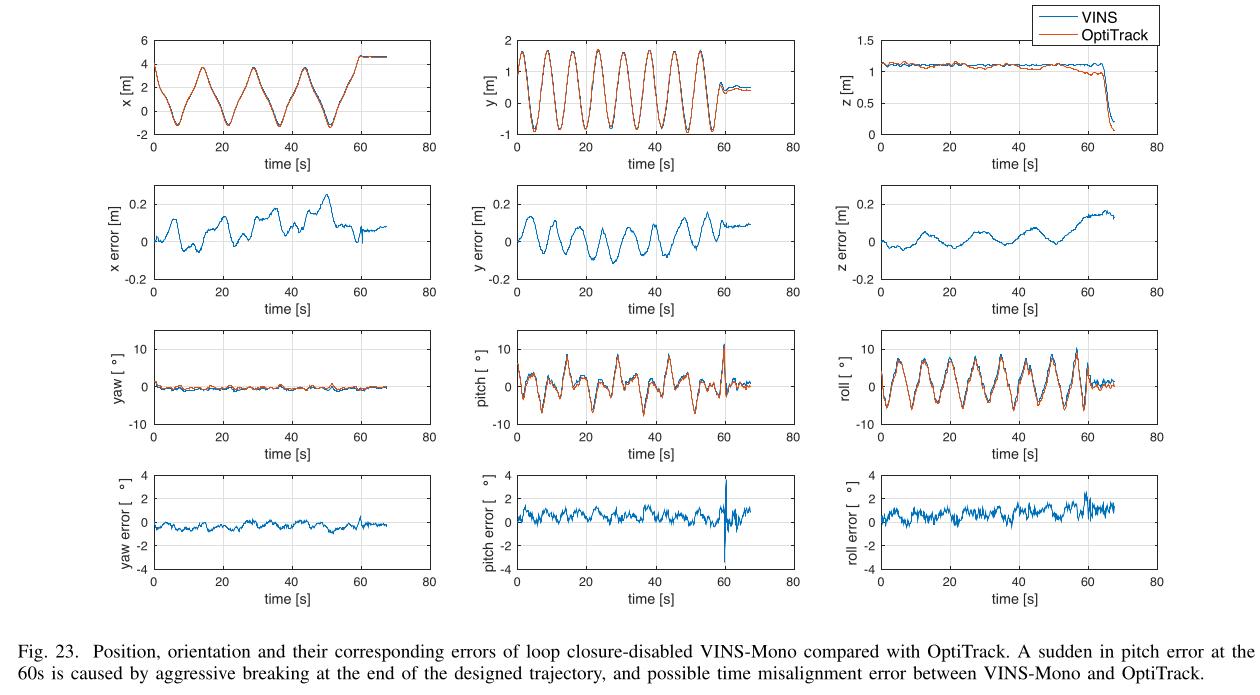# 1. VINS-Mono: A Robust and Versatile Monocular Visual-Inertial State Estimator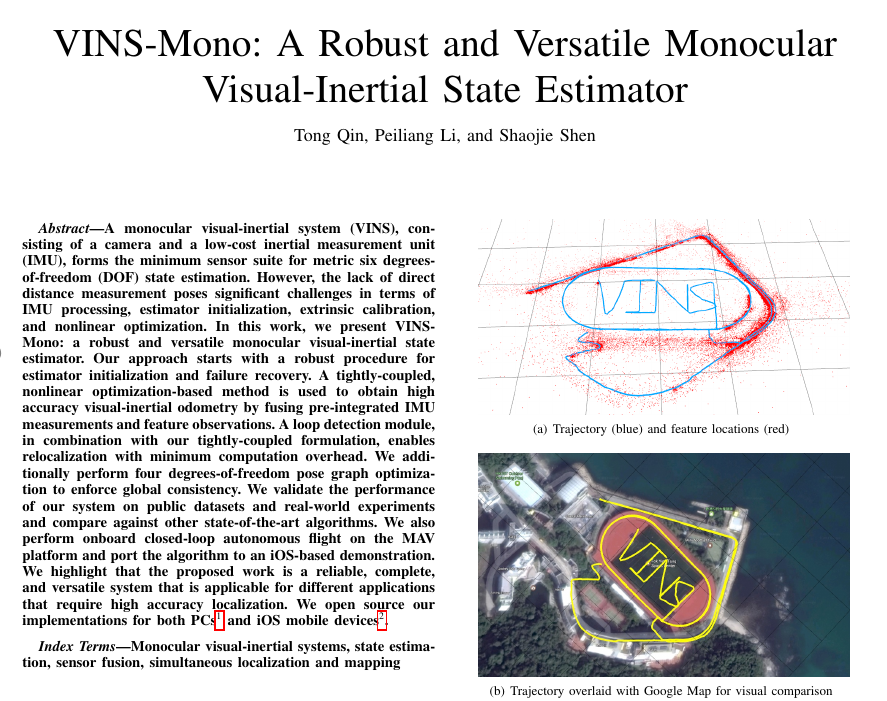# 3. 介绍

1） 初始化

2） 核心处理

3） 回环、重定位

4） 位姿图

5） 小结

• 一个鲁棒的初始化过程，能够从未知的初始状态引导系统
• 种紧密耦合的、基于优化的单目视觉惯性测程方法，具有摄像机-IMU外部校准和IMU偏置估计功能
• 在线回环检测以及紧耦合的重定位
• 四自由度的位姿图优化
• 用于无人机导航、大规模定位和移动AR应用的实时性能演示
• 与ROS完全集成的PC版本和运行在iPhone6s或以上的iOS系统的开源版本

。。。

# 5. 系统概述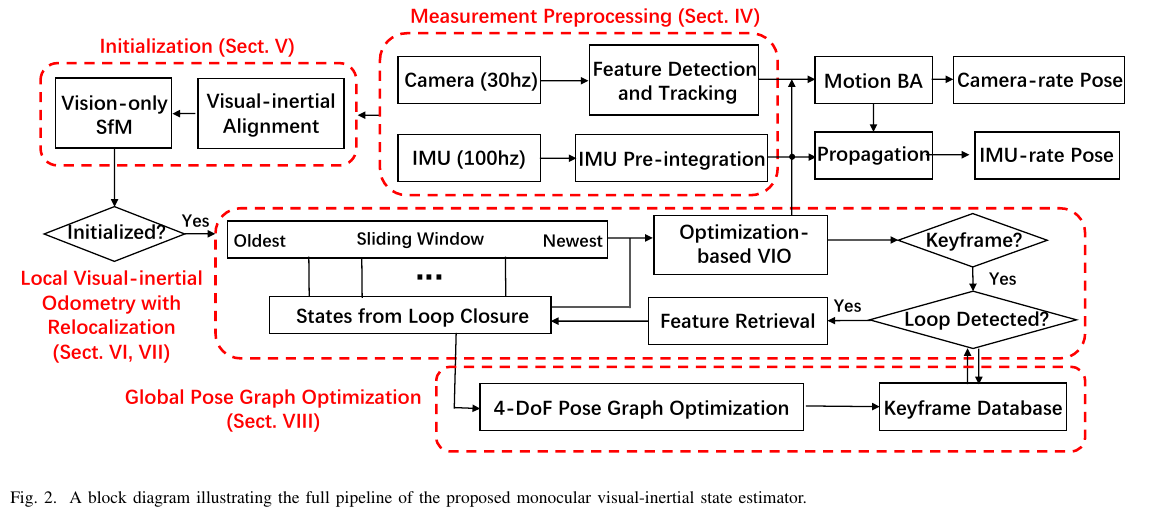VIO与重定位模块紧密地融合了IMU测量的预积分特征观测从回环中重新检测的特征

• $$(\cdot)^w$$表示世界坐标系(导航坐标系)
• 重力g的方向与世界坐标系的z轴对齐，$$g^w=[0,0,g]^T$$代表了世界坐标系的重力向量
• $$(\cdot)^b$$是机体坐标系，也定义为IMU的坐标系
• $$(\cdot)^c$$是相机坐标系
• 使用旋转矩阵$$R$$和哈密顿四元数$$q$$来表示旋转(我们主要在状态向量中使用四元数，但旋转矩阵也用于方便地旋转三维向量)
• $$q_b^w,p_b^w$$代表了从body坐标系到world坐标系的旋转和平移变换
• $$b_k$$是在第k帧图片的机体坐标系
• $$c_k$$是在第k帧图片的相机坐标系
• $$\otimes$$代表了两个四元数之间的乘法运算
• $$(\hat{\cdot})$$用来表示噪声观测或者是不确定性的估计

## 5.1. 测量预处理

• 对于视觉测量，我们跟踪连续帧之间的特征，并在最新帧中检测新特征
• 对于IMU测量，我们预积分两帧之间的数据（注意，我们使用的低成本IMU的测量受到偏差和噪声的影响。因此，我们特别在IMU预积分过程中考虑了bias）

### 5.1.1. 视觉前端处理

• 一是与前一帧的平均视差 (如果当前帧和最新关键帧 跟踪到的特征点的平均视差超过了阈值范围，就认为当前帧可作为新的关键帧，需要注意的是，这里不仅仅是平移，旋转也可以导致视差的变化)。另外，如果仅有旋转，那么跟踪得到的特征点无法完成三角化过程，为了避免这种情况，在计算视差时，我们使用陀螺测量的短期积分来补偿旋转。

注意：这个旋转补偿仅用于关键帧的选取，不包含在VINS公式的旋转项中，最后，尽管IMU角速度测量包含了较大的噪声或者收到bias的影响，这只会导致次优的关键帧选取结果，并不会直接影响最终估计的质量。

• 另外一个准则是: 跟踪质量，如果跟踪的特征点数量低于阈值，那么这一帧会作为新的关键帧，这个标准是为了避免完全丧失特征的情况

### 5.1.2. IMU预积分

IMU预积分首先被提出，使用欧拉角形式对旋转误差进行参数化。

IMU的原始角速度和加速度测量值$$\hat{w},\hat{a}$$可以表示为如下: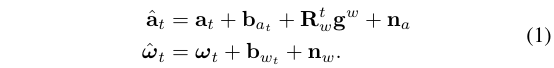IMU测量值，是在body系下的，结合了重力机体的动态加速度bias角速度bias噪声。我们假设，额外的噪声项是高斯噪声，即$$n_a \sim \mathcal{N}(0,\sigma_a^2), n_w \sim \mathcal{N}(0,\sigma_w^2)$$。加速度bias和角速度bias使用随机游走模型，也可以使用高斯分布来表示，即$$n_{ba}\sim \mathcal{N}(0,\sigma_{ba}^2),n_{bw}\sim\mathcal{N}(0,\sigma_{bw}^2)$$，并且有: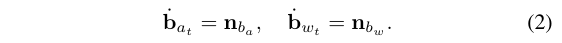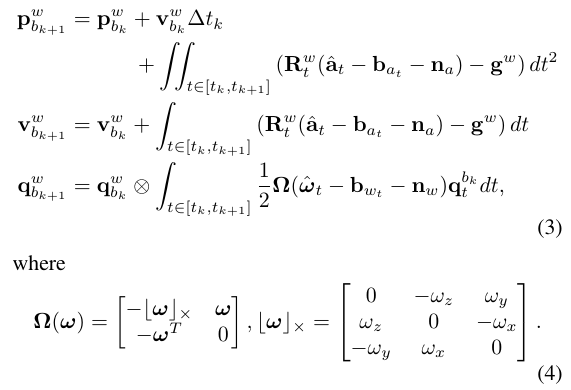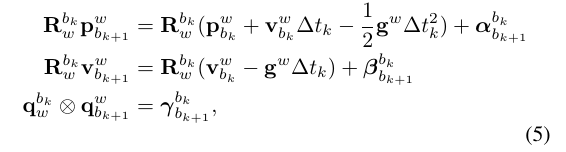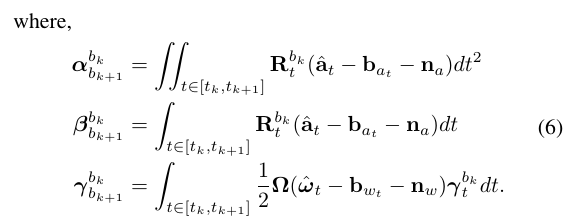• $$R_{w}^{b_{k}}$$: 将数据从世界坐标系转换到k时刻的机体坐标系的旋转变换
• $$R_{w}^{b_k} p_{b_{k+1}}^{w}$$指的是将k+1时刻在世界坐标系下的机体位置转换到k时刻的机体坐标系$$b_k$$后得到的东西
• $$p_{b_{k+1}}^{w}$$指的是将k+1时刻在世界坐标系下的机体位置
• $$R_{w}^{b_k} v_{b_{k+1}}^w$$是k+1时刻在世界坐标系下的机体速度转换到k时刻的机体坐标系$$b_k$$后得到的东西
• $$v_{b_{k+1}}^w$$是k+1时刻在世界坐标系下的机体速度
• $$q_{w}^{b_k}$$是k时刻世界坐标系下的机体姿态，也就是从世界坐标系转换到k时刻的机体坐标系的旋转变换
• $$R_{t}^{b_k}$$是指在$$[t_k,t_{k+1}]$$内的某个时刻t，机体相对于k时刻的机体坐标系的姿态，即表示从时刻 $$t \in [t_k,t_{k+1}]$$k时刻的机体坐标系的旋转变换
• $$\alpha_{b_{k+1}}^{b_{k}}$$指相对于k时刻机体坐标系的位置积分，即这个位置积分量是在k时刻的机体坐标系$$b_{k}$$下的
• $$\beta_{b_{k+1}}^{b_{k}}$$指相对于k时刻机体坐标系的速度积分，即这个速度积分量是在k时刻的机体坐标系$$b_{k}$$下的

$$\alpha_{b_{k+1}}^{b_k},\beta_{b_{k+1}}^{b_k},\gamma_{b_{k+1}}^{b_k}$$与IMU的偏置$$b_{at},b_{wt}$$有关，然而这个偏置变化一般较小，如果变化了，我们可以使用一阶近似，然后重新计算预积分，否则，预积分只需计算一次就可以了{这里说的操作，对应于式(12)}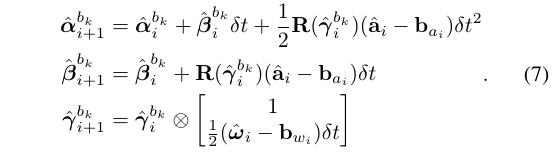• $$i$$表示在$$[t_k,t_{k+1}]$$时间段内的离散化时刻，(即两帧图像之间有很多个时刻的IMU测量值)
• $$\delta t$$是IMU测量间隔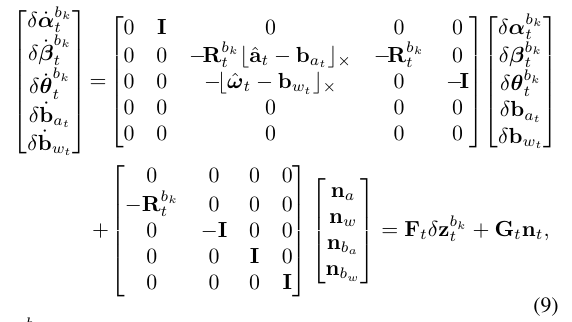$$P_{b_{k+1}}^{b_k}$$可以使用离散时间的一阶近似进行递归计算得到，初始化协方差$$P_{b_{k}}^{b_k}=0$$: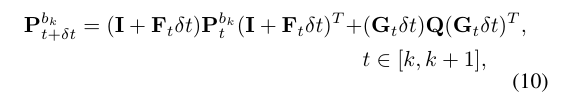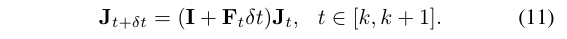$$\alpha_{b_{k+1}}^{b_k},\beta_{b_{k+1}}^{b_k},\gamma_{b_{k+1}}^{b_k}$$相对于bias的一阶近似可以写成: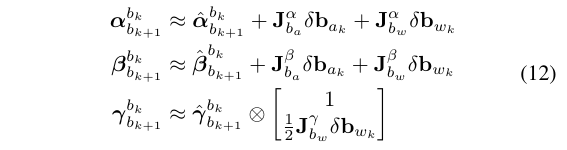• $$J_{b_a}^\alpha$$$$J_{b_{k+1}}$$的子块，其位置与$$\frac{\delta \alpha_{b_{k+1}}^{b_k}}{\delta b_{a_{k}}}$$相对应
• 对于$$J_{b_w}^\beta,J_{b_a}^\beta,J_{b_{w}}^\beta,J_{b_{w}}^\gamma$$也同样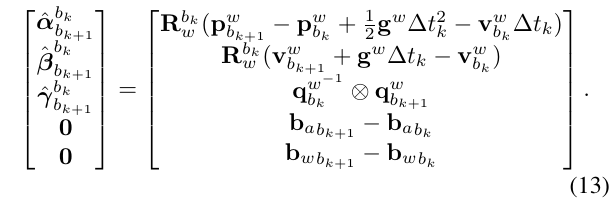## 5.2. 初始化

### 5.2.1. 滑动窗口：Vision-Only SFM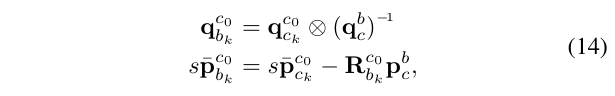### 5.2.2. VI对齐

#### 5.2.2.1. Gyro Bias标定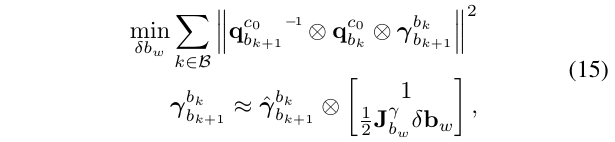• $$\mathcal{B}$$是滑动窗口中所有帧的集合
• 通过使用前面推导的bias雅克比，我们有$$\hat{\gamma}_{b_{k+1}}^{b_k}$$关于gyro bias的一阶近似
• 在这种方式下，我们获得了关于gyro bias的初始标定
• 然后我们使用新的gyro bias值，对IMU的预积分项$$\alpha_{b_{k+1}}^{b_k},\beta_{b_{k+1}}^{b_k},\gamma_{b_{k+1}}^{b_k}$$进行重传播

#### 5.2.2.2. 速度、重力向量、尺度的初始化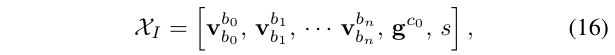• $$v_{b_k}^{b_k}$$是在机体坐标系$$b_k$$的速度
• $$g^{c_0}$$是在参考坐标系$$c_0$$坐标系的重力向量
• $$s$$是单目sfm的尺度参数，以米为单位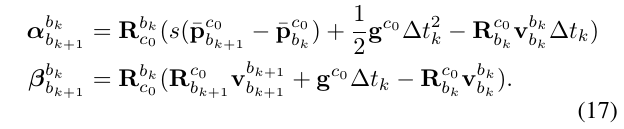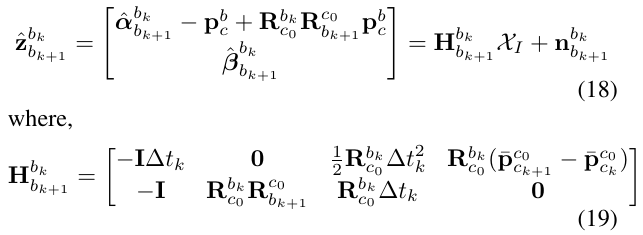• $$R_{b_{k}}^{c_0},R_{b_{k+1}}^{c_0},\bar{p}_{c_k}^{c_0},\bar{p}_{c_{k+1}}^{c_0}$$都是从单目sfm中获取到的值
• $$\Delta t$$是两个连续图像帧之间的时间间隔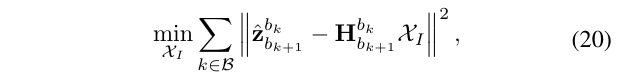#### 5.2.2.3. 重力对齐

• $$g$$是已知重力的量级
• $$\bar{\hat{g}}$$是代表重力方向的单位向量
• $$b_1,b_2$$是两个正交基章程的正切平面，如图5所示
• $$w_1,w_2$$是对应于$$b_1,b_2$$两个基的位移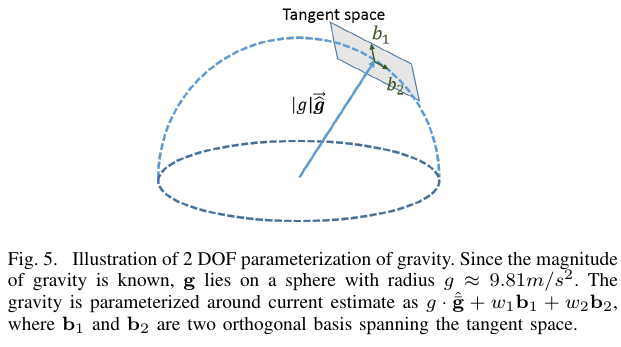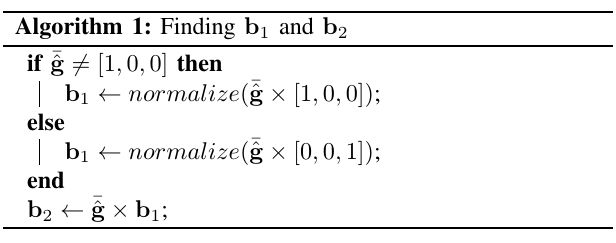## 5.3. 紧耦合VIO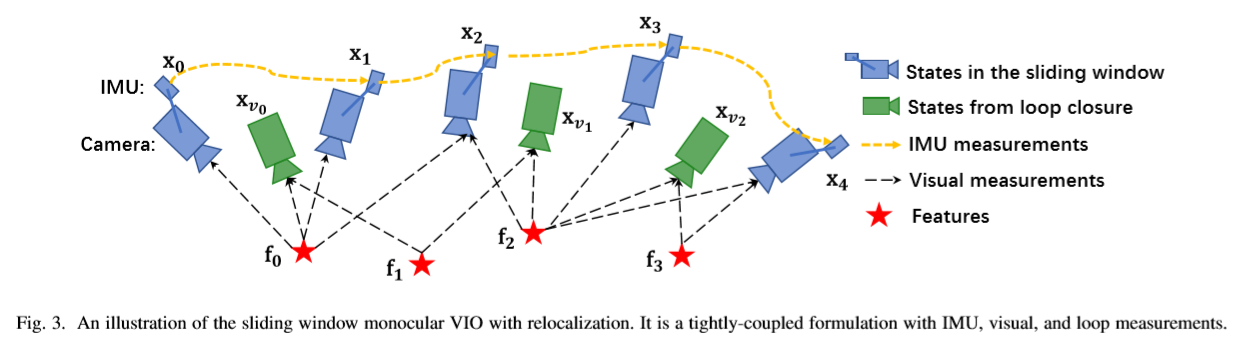### 5.3.1. 系统状态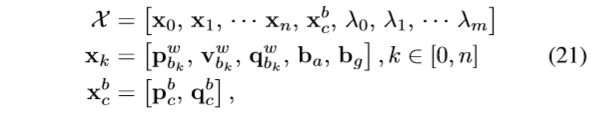• $$x_k$$是IMU在第k帧图像对应的时刻的状态，包含了IMU（机体）在世界坐标系下的位置、速度和姿态，以及在机体坐标系下的IMU加速度bias和角速度bias
• n表示所有的关键帧数量
• m表示滑动窗口内所有的特征点的数量
• $$\lambda_l$$表示第l个特征点相对于其第一次观测的逆深度值

• 先验项
• 视觉重投影残差项
• IMU预积分残差项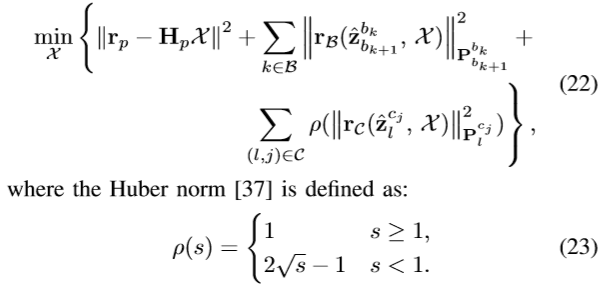• $$r_{B}(\hat{z}_{b_{k+1}}^{b_k},X)$$表示IMU的测量残差
• $$r_c(\hat{z}_{l}^{c_j},X)$$表示视觉测量残差
• $${B}$$是IMU测量数据的集合
• $${C}$$是当前滑动窗口中至少被观测到两次或以上的特征点集合
• $${r_p,H_p}$$是来自边缘化得到的先验信息

### 5.3.2. IMU测量误差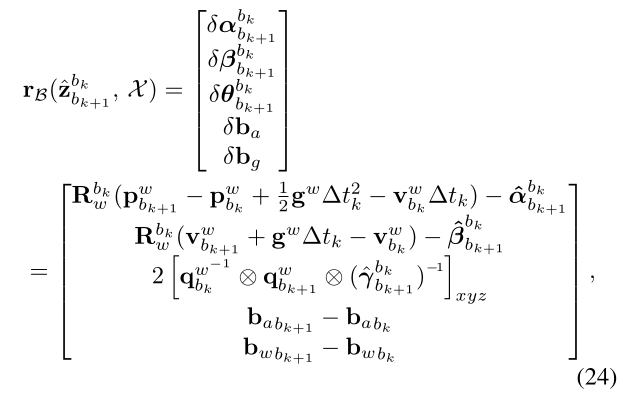• $$[\cdot]_{xyz}$$提取四元数中的角度部分，作为误差状态的表达，即$$\delta\theta_{b_{k+1}}^{b_k}$$是关于四元数的误差状态
• $$[\hat{\alpha}_{b_{k+1}}^{b_k},\hat{\beta}_{b_{k+1}}^{b_k},\hat{\gamma}_{b_{k+1}}^{b_k}]^T$$是关于IMU的在两个图像帧之间的预积分项，这一项仅与包含噪声的加速度和角速度测量有关
• 加速度计和陀螺仪的bias被包含在參差项中，用以实现在线的矫正

### 5.3.3. 视觉测量误差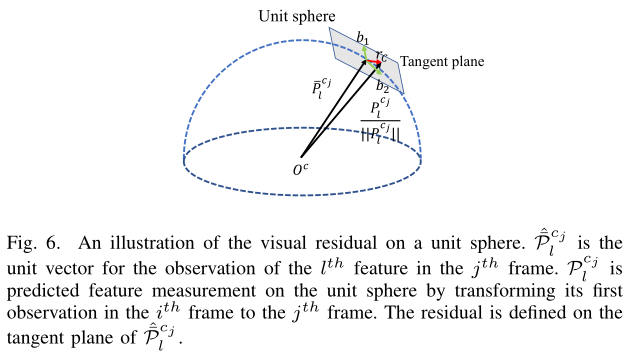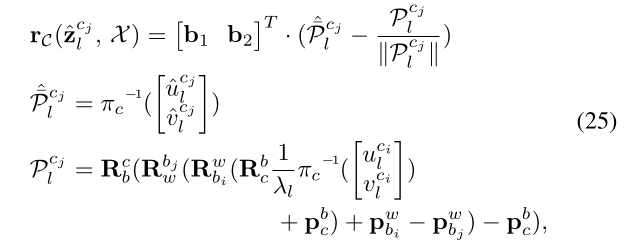• $$[u_l^{c_i},v_l^{c_i}]$$表示第l个特征的第一次被第i帧图像观测所在的像素位置
• $$[u_l^{c_j},v_l^{c_j}]$$表示第l个特征被第j帧图像观测所在的像素位置
• $$\pi_c^{-1}$$表示使用相机模型的内参，将像素点坐标反投影成一个单位向量（3D点）
• $$P_l^{c_j}$$用于(式22)，是在正切空间中一个固定长度的标准协方差
• $$\hat{\bar{\mathcal{P}}}_l^{c_j}$$是将第j帧观测到第l个特征点的像素坐标反投影得到的3D向量
• $$\mathcal{P}_l^{c_j}$$是将第i帧观测到第l个特征点的像素坐标 1）先反投影成3D向量(归一化平面上的) 2）再利用逆深度，得到在第i帧相机坐标系下的3D点 3）然后根据IMU和相机的外參，将该3D点变换到IMU坐标系下 4）根据(第i帧)待估计的机体位姿$$T_{b_i}^{w}$$，将该点变换到世界坐标系下 5）根据(第j帧)待估计的机体位姿$$[T_{b_j}^{w}]^{-1}$$，将该点投影到第j帧机体坐标系下 6）然后，再次根据IMU和相机外參，将该点转换到第j帧的相机坐标系下

### 5.3.4. 边缘化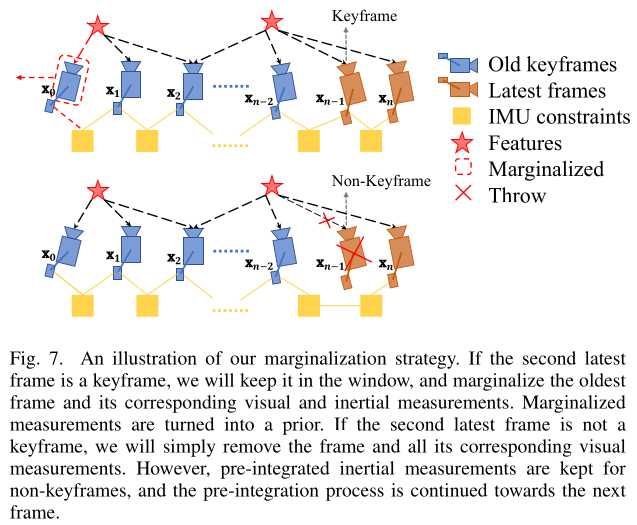• 如果是关键帧，那么将其插入到滑动窗口中，同时，弹出滑动窗口中排在最前面（最old的）的那一帧，并且对其视觉和IMU测量信息进行边缘化，转化为先验矩阵$$H_{prior}$$
• 如果不是关键帧，那么对于这个最新的一帧，不插入到滑动窗口中，并且舍弃其视觉信息，保留IMU的测量信息（因为需要其预积分信息来进行向前推算）

### Motion-only BA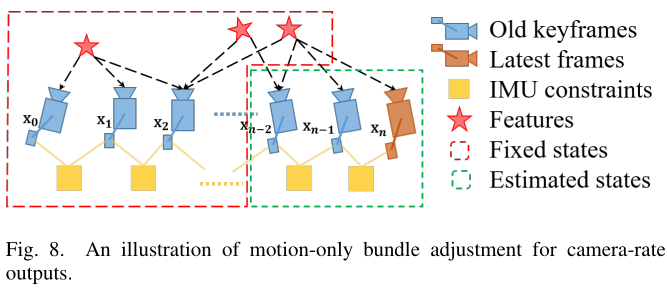### 故障诊断和恢复

• 在最新帧中被跟踪的特征数小于某个阈值
• 估计器的最后两次输出（位置、姿态）有较大的不连续性
• bias或外參估计产生了较大的变化

## 重定位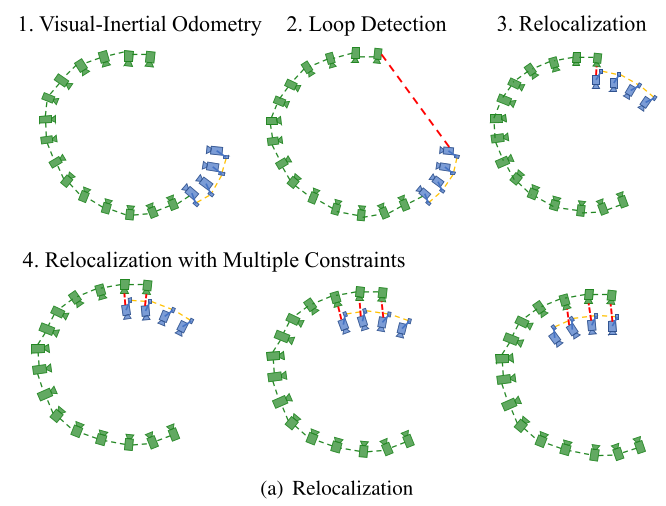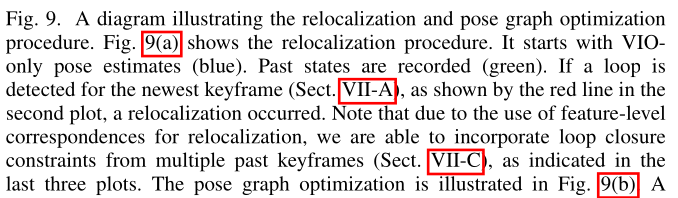### 特征恢复

• 2D-2D： 使用RANSAC进行基础矩阵F的测试，对当前帧图像的2D观测点与回环候选帧图像（实际上是特征点）进行基础矩阵F测试
• 3D-2D： 使用RANSAC的PnP测试，基于当前滑动窗口中已知的3D点，以及回环候选帧图像中的2D特征点，进行PnP测试

### 紧耦合的重定位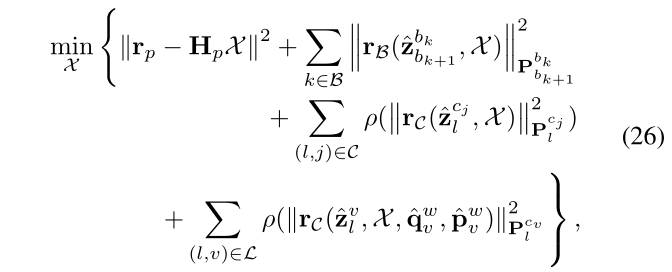• $$\mathcal{L}$$是从闭环关键帧与当前滑动窗口恢复出来的特征关联的集合
• $$(l,v)$$表示在闭环关键帧中观测到的第l个特征点

## 全局Pose Graph优化

### 向Pose Graph中添加关键帧

• 序列边：一个关键帧会与之前的几个关键帧建立起一些sequential edge（序列边）。一条序列边代表了两个关键帧的相对变换关系，这个相对变换可以直接从VIO获取，考虑一个较的关键帧i和其前一帧j，那么这个序列边仅仅包含相对位置$$\hat{p}_{ij}^i$$和相对yaw角$$\hat{\psi}_{ij}$$: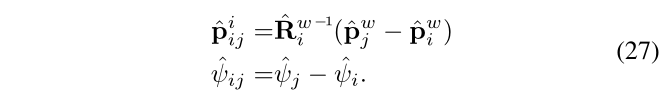• 回环边：如果这个被边缘化的关键帧与之前的回环帧建立了回环连接，那么这个回环连接同样被加入到Pose Graph中。相似的，回环边也仅仅包含4自由度的相对关系，如上面的(式27)所示，这个回环边的数据来自重定位得到的结果

### 4自由度的Pose Graph优化• $$\hat{\phi}_i,\hat{\theta}_i$$分别是roll和pitch的估计值，可以直接从单目VIO状态估计器中直接获取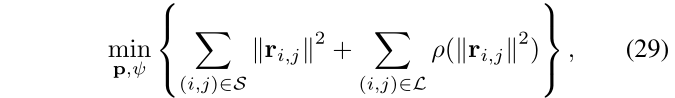• $$\mathcal{S}$$代表所有序列边
• $$\mathcal{L}$$代表所有回环边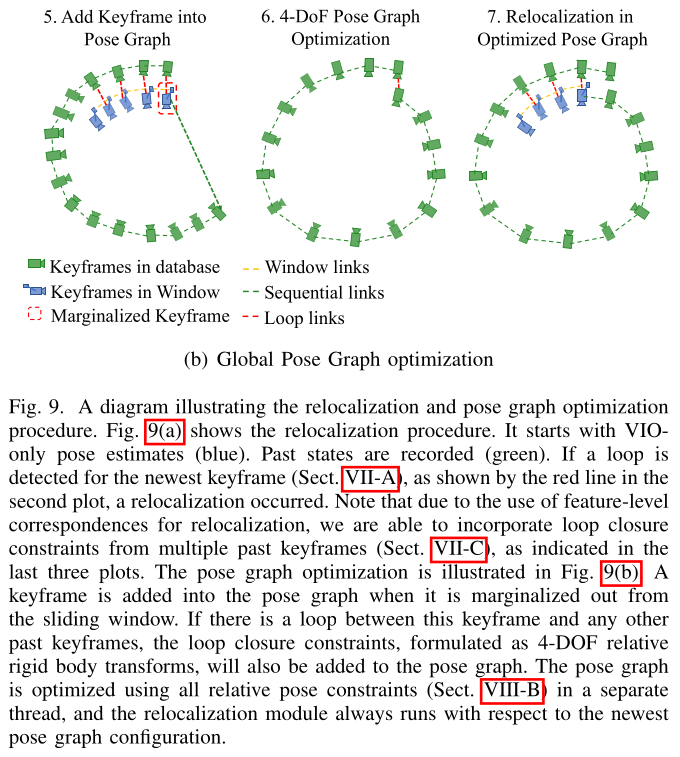## 实验

### 公开数据集测试对比

• MH_03_median
• MH_05_difficult

• VINS表示仅仅是VIO（视觉-惯性里程计）(没有回环部分)
• VINS_loop表示带有完整版的重定位和位姿图优化的VINS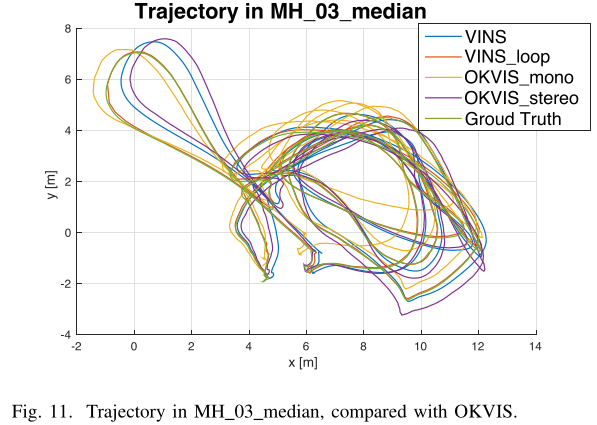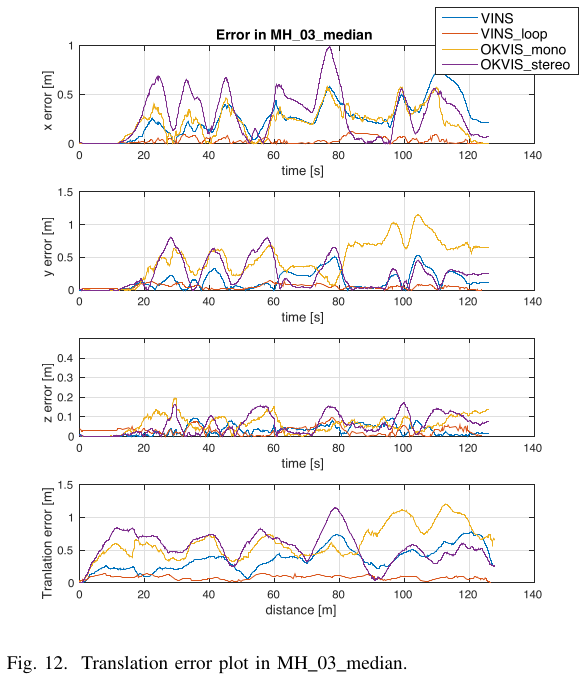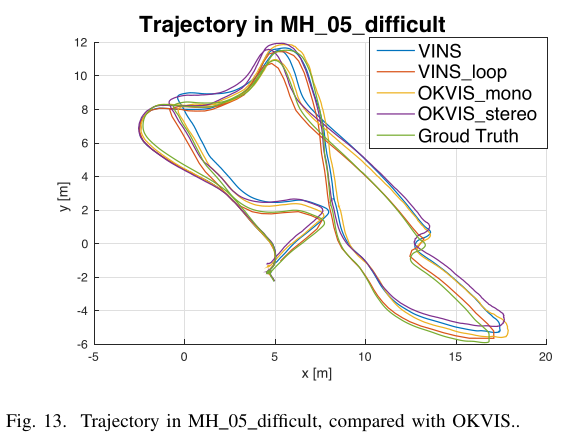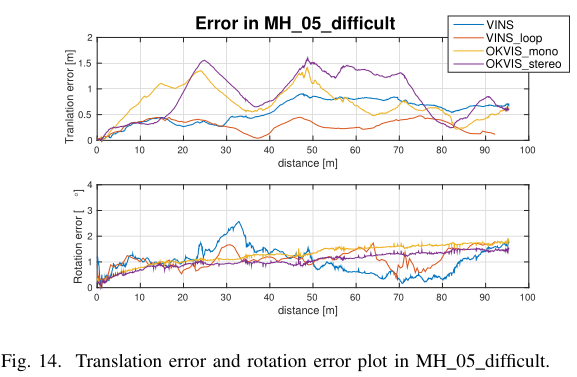rollpitch角的估计上，OKVIS的估计更加准确一些，一个可能的原因是VINS-Mono使用的预积分，这是使用一阶近似来进行IMU的传播，以节省计算资源。

### 室内环境对比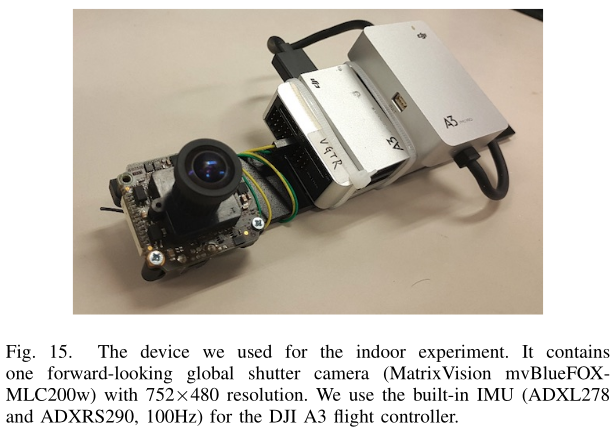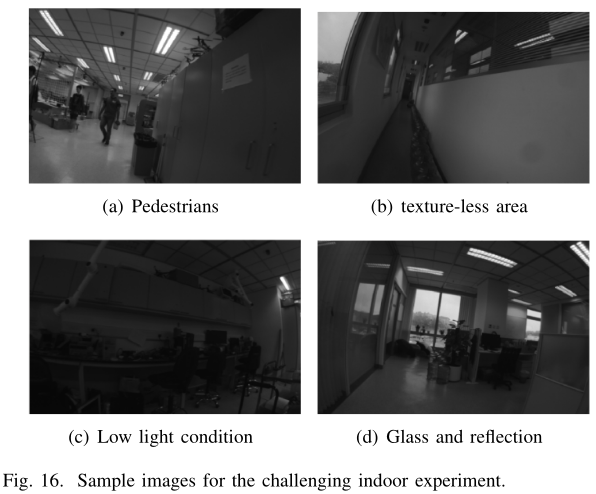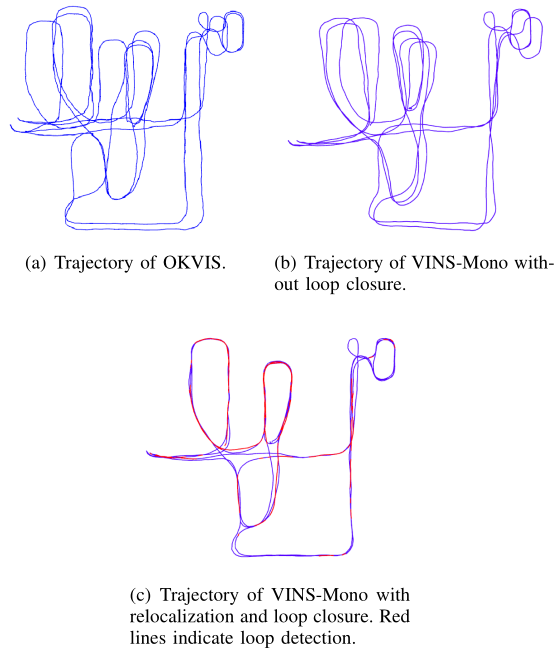### 大范围环境测试

#### 实验室外面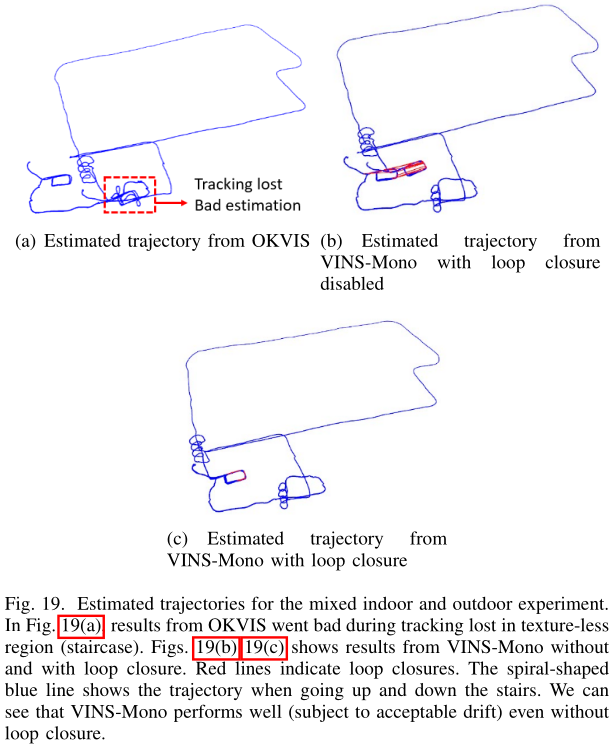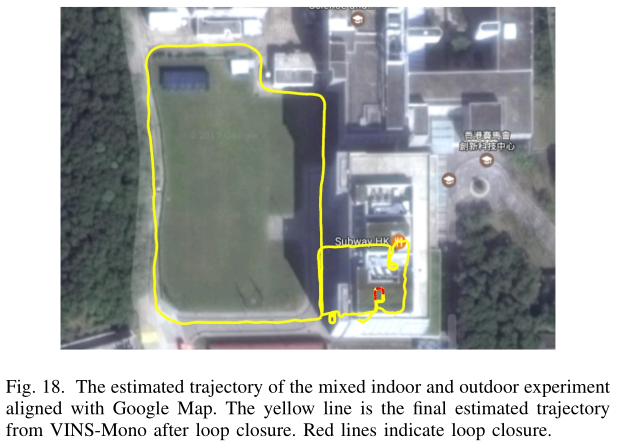OKVIS的最终漂移是[13.80，-5.26，7.23]米，分别在x、y和z轴上。无回环的VINS-Mono的最终漂移是[-5.47, 2.76,-0.29]米，其占轨迹长度的0.88%，小于OKVIS的2.36%，带有回环功能的VINS-Mono，最终的漂移是[-0.032, 0.09, -0.07]米，对于整条轨迹长度而言，是微不足道的。

#### 绕校园环境测试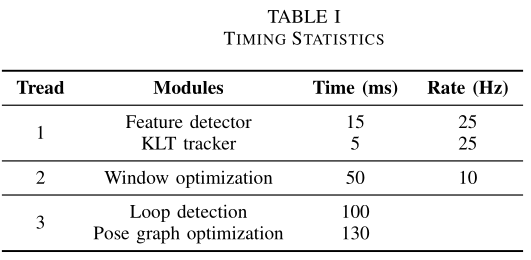#### 应用1：无人机的反馈控制